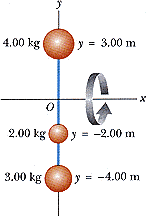# Find the moment of inertia about the x axis

## Homework Statement

Rigid rods of negligible mass lying along the y axis connect three particles (Fig. P10.20). The system rotates about the x axis with an angular speed of 2.90 rad/s.Figure P10.20

(a) Find the moment of inertia about the x axis.
______kgm2
Find the total rotational kinetic energy evaluated from 1/2 I2.
______J
(b) Find the tangential speed of each particle.
______m/s (4.00 kg particle)
______m/s (2.00 kg particle)
______m/s (3.00 kg particle)
Find the total kinetic energy evaluated from mi vi2/2.
______J

## Homework Equations

I = sum of (r^2*m)

## The Attempt at a Solution

My teacher told us to use I = sum of (r^2 * m) i am not sure how this works if some of the r's (distances from the axis of rotation) are negative. i tried subtracting the negative ones, or just plugging them in and squaring them, thus making them positive, to no avail. Any help would be greatly appreciated! the most difficult part of this for me is the moment of inertia.

Last edited:

## Answers and Replies

Hootenanny
Staff Emeritus
Science Advisor
Gold Member
What are you struggling with?

finding the moment of inertia of the structure, after that i think i should be able to handle it

Hootenanny
Staff Emeritus
Science Advisor
Gold Member
So you've done something like this;

$$I = 4(3)^2 + 2(-2)^2 + 3(-4)^2$$

Is that correct?

So you've done something like this;

$$I = 4(3)^2 + 2(-2)^2 + 3(-4)^2$$

Is that correct?

yup, i did exactly that...didn't get it right...

oh wow, never mind, i made some type of calculation mistake. for some reason i got 140 when it shoudl have been 92. my last question is about the last part, it says to find the total kinetic energy evaluated from (sum of)mi vi^2/2.